# How to use the SYD Function in Excel

SYD function is “Sum of Years” declining depreciation is the way of calculating the depreciation of any asset for each period. “Sum of Years” declining balance method is the way where the total sum of years becomes a decline factor of depreciation of an asset. The below formula is the way to find the “Sum of Years” decline in depreciation.The SYD function returns depreciation decline amount by taking sum of years data for the specific product.
Syntax:

=SYD (cost, salvage, life, period)

Cost : Cost price of the asset
Salvage : remaining price of the asset after its lifetime period
Life : period after which cost price becomes salvage price
period : specific period for which we need to calculate depreciation.
Let’s understand this function using it in an example.
Here we took a product as Iphone. The cost price & salvage price of the latest Iphone is shown in the snapshot below.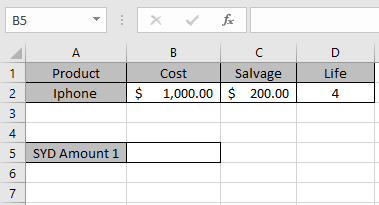Now we need to calculate the depreciation amount for the Iphone.
Use the formula to calculate depreciation for the first year:

=SYD (B2, C2, D2, 1)

B2 : Cost price of the asset
C2 : remaining price of the asset after a period
D2 : 4 years after cost price becomes salvage price
1 : first year to calculate depreciation amount.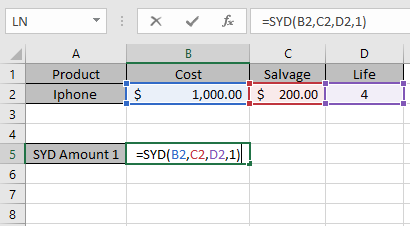The values to the formula are taken as cell reference.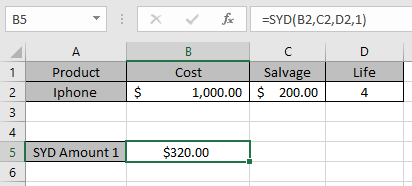As you can see from the above returned value, first year \$ 320 will depreciated from the asset cost value.
Now we will calculate the depreciation amount for each year separately.
Use the formula to calculate depreciation for second year:

=SYD (B2, C2, D2, 2)

B2 : Cost price of the asset
C2 : remaining price of the asset after a period
D2 : 4 years after cost price becomes salvage price
2 : first year to calculate depreciation amount.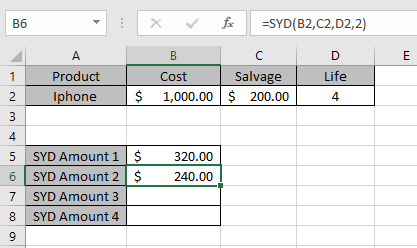Similarly calculate the depreciation for Third & Fourth year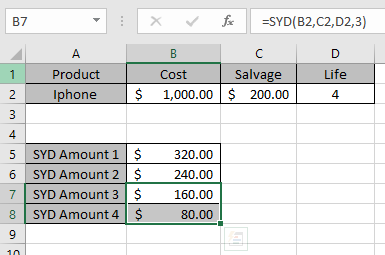So, we got different depreciation for each period

 Year Depreciation Salvage value 0 0 \$ 1000 1 \$ 320.00 \$ 680 2 \$ 240.00 \$ 440 3 \$ 160.00 \$ 280 4 \$ 80.00 \$ 200

This is the drop depreciation table for the asset.
SYD function shows that depreciation reduces as factor which is “Sum of Years”.
You can see from the above table that depreciation amount is deducted from the salvage amount for every year up till life of an asset.
Note:

1. The function generates #VALUE! Error if arguments to the function is non - numeric.
2. The function generates #NUM! Error if:
• The value of cost and salvage is less than 0.
• The value of life of asset is less than or equals to zero.
• The period argument is less than or equals to 0 or greater than life period.

Hope you understood how to use SYD function and referring cell in Excel. Explore more articles on Excel  financial functions here. Please feel free to state your query or feedback for the above article.

Related Articles

How to use the VDB Function in Excel

How to use the DB Function in Excel

How to use the DDB Function in Excel

Popular Articles:

50 Excel Shortcuts to Increase Your Productivity

How to use the VLOOKUP Function in Excel

How to use the COUNTIF in Excel 2016

How to use the SUMIF Function in Excel

Terms and Conditions of use

The applications/code on this site are distributed as is and without warranties or liability. In no event shall the owner of the copyrights, or the authors of the applications/code be liable for any loss of profit, any problems or any damage resulting from the use or evaluation of the applications/code.